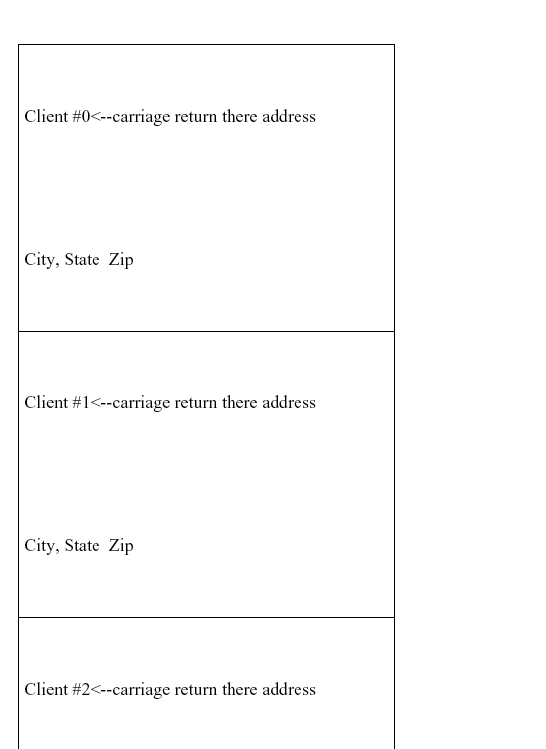Categories

## FPDF.asp carriage return / line feed in MultiCell

Recently I was given the task of printing address labels from a database using Avery 5160 labels. These labels are 3 columns of 10 rows. 2.625″ x 1″ (66.6mm x 25.4mm).

I was having trouble with carriage returns. First problem is that the ASP version of FPDF doesn’t seem to recognize the \n line feed. It was easy enough to replace it with Chr(10) but I suppose I had a misconception of how FPDF handles line feeds in that it used the entire height of the multicell height for every line of text.

At first I used this incorrect code to test (notice the carriage return has no effect):

```<%@language=vbscript%>
<!--#include file="fpdf.asp"-->
<%
Dim i,pdf
Set pdf=CreateJsObject("FPDF")
pdf.CreatePDF "P","cm","Letter"
pdf.SetPath("fpdf/")
pdf.SetTopMargin(1.27)
pdf.SetLeftMargin(.42)
pdf.SetRightMargin(.42)
pdf.Open()
pdf.SetFont "Times","",9
for i=0 to 40
labelText = "Client #" & i & Chr(13) & "<--carriage return there address" & Chr(10) & "City, State  Zip "
pdf.MultiCell 6.66,2.54,labelText,1,L
Next
pdf.Close()
pdf.Output()
Set pdf = Nothing
%>```

with the results shown below:Then after googling a bit I found a php version of a script for creating a PDF for Avery 5160 labels and ported it to ASP:

```<%@language=vbscript%>
<!--#include file="fpdf.asp"-->
<%
Set pdf = CreateJsObject("FPDF")
pdf.CreatePDF "P","mm","Letter"
pdf.Open()
pdf.SetFont "Arial","B",10
pdf.SetMargins 0,0
pdf.SetAutoPageBreak(0)
x = 0
y = 0
for a = 1 to 40
' Make label text
LabelText = "Contact Name" & a & chr(10) & "Entire Company Name" & a & chr(10) & "1313 Mockingbird Lane" & chr(10) & "Chicago, IL  60617"
LeftMargin = 4.2
TopMargin = 12.7
LabelWidth = 66.6
LabelHeight = 25.45
' Create Co-Ords of Upper left of the Label
AbsX = LeftMargin + ((LabelWidth + 4.22) * x)
AbsY = TopMargin + (LabelHeight * y)
pdf.SetXY AbsX+3,AbsY+3
pdf.MultiCell LabelWidth-8,4.5,LabelText,0,L
y=y+1
if (y=10) then
x=x+1
y = 0
if (x = 3) then
x = 0
y = 0